﻿Standard Deviation

# Standard Deviation

The standard deviation (StandardDeviation) (std) is a statistic that measures the dispersion of a dataset relative to its mean and is calculated as the square root of the variance (Variance). Standard deviation is a statistical measurement in finance that, when applied to the annual rate of return of an investment, sheds light on the historical volatility of that investment. The greater the standard deviation of a security, the greater the variance between each price and the mean, which shows a larger price range.Calculation

In the case of a set of N values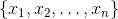and the arithmetic mean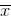, the sample (unbiased) standard deviation is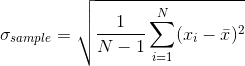The population standard deviation is differ only in division factor: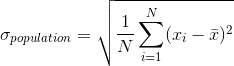Chart Example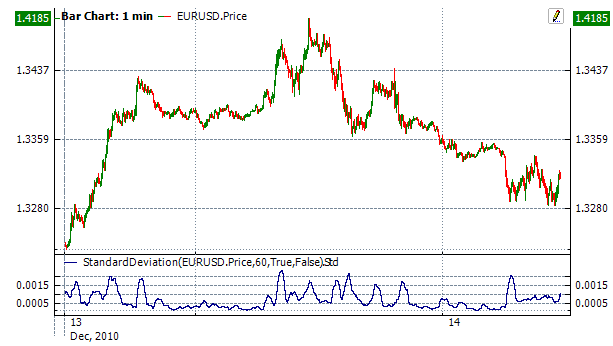Implementation and Usage

To initialize indicator, use one of the following constructors:

StandardDeviation – set default values: period = 14

StandardDeviation(Int32, Boolean) – set value for period

StandardDeviation(TimeSpan, Boolean) – sets time period

Use

Std - property to get current valueExample
C#
``` 1// Create new instance
2var indicator = new StandardDeviation(28);
3
4// Number of stored values
5indicator.HistoryCapacity = 2;
6